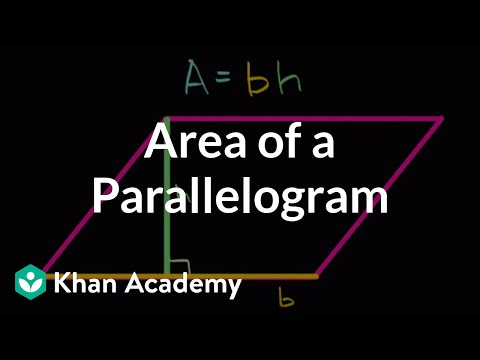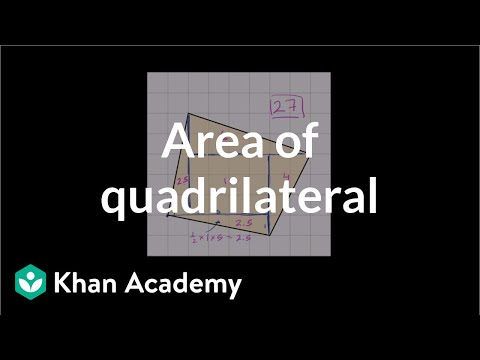Video

# Area of trapezoids (Full video)

Description: Area of a trapezoid is found with the formula, A=(a+b)/2 x h. Learn how to use the formula to find area of trapezoids. Created by Sal Khan. So what would we get if we multiplied this long base 6 times the height 3? The area of a figure that looked like this would be 6 times 3.

### Other videos you might be interested in### Area of a parallelogram (Full video)